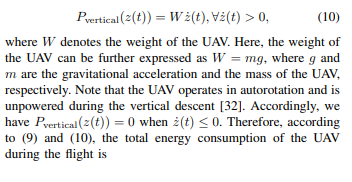# Do work upwards, but do not do downwards work how to represent energy in cvx？Do work upwards, but do not do downwards work how to represent energy in cvx？
That is, the drone only needs to do work and consume energy when it is rising, and when it is falling, it does not consume energy because of its own gravity, which is 0 joules, so I want to ask how to express this in cvx? What I currently write is EZ=mg.*max((Zn(n+1) - Zn(n)),0), mg means gravity, Zn means height。

If you have a convex optimization problem, forum readers might be able to help you enter it in CVX. This forum is not the appropriate place for help formulating an optimization problem to model UAVs.

CVX does have the following built-in capability, which you can use as a building block.

help pos

pos Positive part.
pos(X) = MAX(X,0). X must be real.

`````` Disciplined convex programming information:
pos(X) is convex and nondecreasing in X. Thus when used in CVX
expressions, X must be convex (or affine).``````